Electrical Measurement homework Help at TutorEye

Best Homework Help For Electrical Measurement

Electrical Measurement:

Electrical measurement comprises different devices, methods and mathematical expressions to evaluate electrical quantities and units. It deals in the measurement of various electrical entities such as current, voltage, resistance, capacitance etc. Properties are converted into electrical signals and waves, which are further measured and recorded using different instruments such as ammeter, voltmeter, galvanometer etc either in analog or digital form.

Electrical Measurement Sample Questions:

Question 1: D.C bridges are used for the measurement of _________.

a) capacitance                   b) current

c) resistance                    d) voltage

Explanation: Resistance measurements are used

Question 2: __________ and _________ are connected between the two ends of Wheatstone bridge.

a) Ohmmeter, galvanometer              b) Ammeter, voltmeter

c) Ammeter, galvanometer              d) Galvanometer, battery

Explanation: Galvanometer is connected between opposite

Question 3: Which of the following is the correct condition for a balanced wheatstone bridge?

a)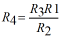b)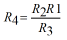c)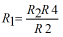d)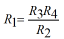Answer:Explanation: The balanced condition of a wheatstone bridge is determined by the ratio of resistor R1 and R2

Question 4: ___________ is source at very low frequency in an A.C. bridge.

a) Electromotive force                   b) Power line

c) Ammeter                                   d) Galvanometer

Explanation: The power line acts as a source of supply

Question 5: High current and high voltages are measured by __________ and _________ respectively.

a) current transformer, potential transformer              b) potential transformer, current transformer

c) primary transformer, secondary transformer          d) none of these

Explanation: Current transformer and potential transformer are used with A.C. ammeter and voltmeter

Question 6: Which of the following is a correct expression for a current transformer’s turns ratio?

a)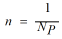b)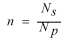c)d)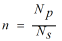Answer:Explanation: Turn ratio for the current transformer is the ratio

Question 7: ______________ is used to obtain a high range of electronic voltmeter in a D.C.

a) Transducer                b) Resistor

c) Attenuator                d) Transformer

Explanation: Attenuator reduces the power of the signal and gets a high range of values

Question 8: Physical changes are sensed by ____________.

a) Electrical transducer              b) Mechanical transducer

c) Both (a) and (b)                     d) None of these

Explanation: Mechanical transducer delivers physical

Question 9: In an oscilloscope, an electron beam is deflected in _________ directions.

a) one                   b) two

c) three                 d) four

Explanation: The electron beam is deflected in two directions

Question 10: IC chips on digital voltmeter are used to ___________.

a) reduce the cost                     b) increase the size

c) increase the resistance         d) increase the cost